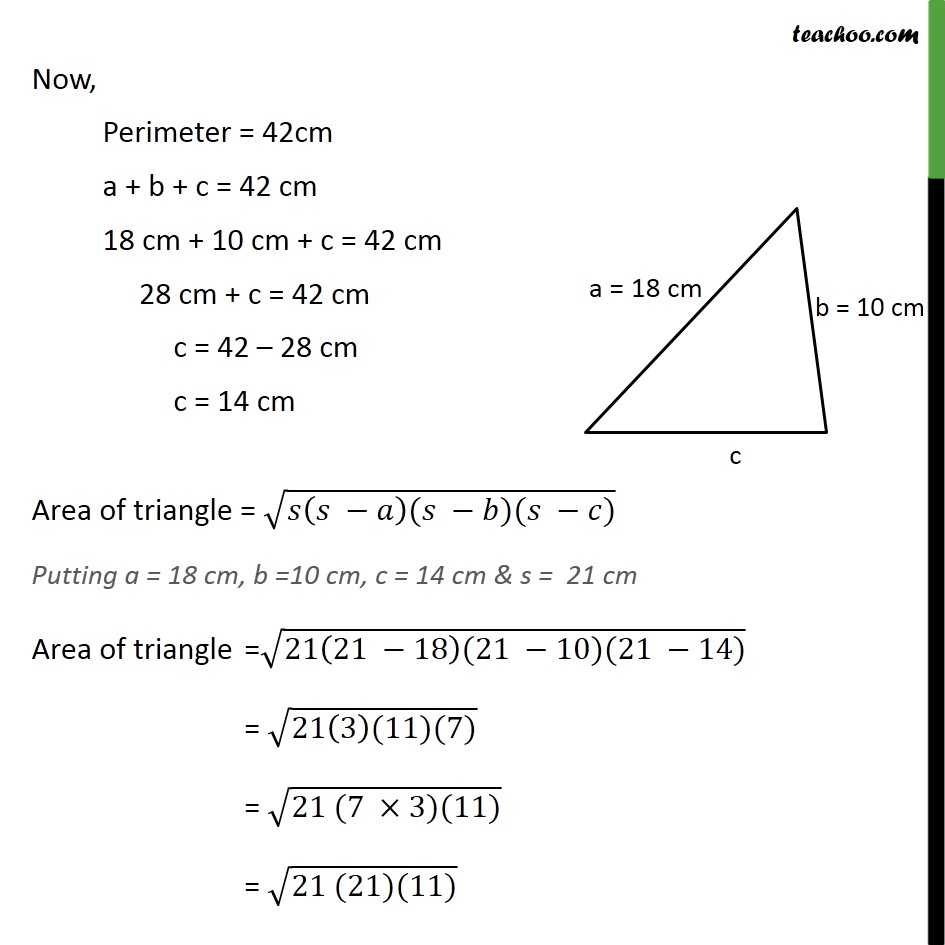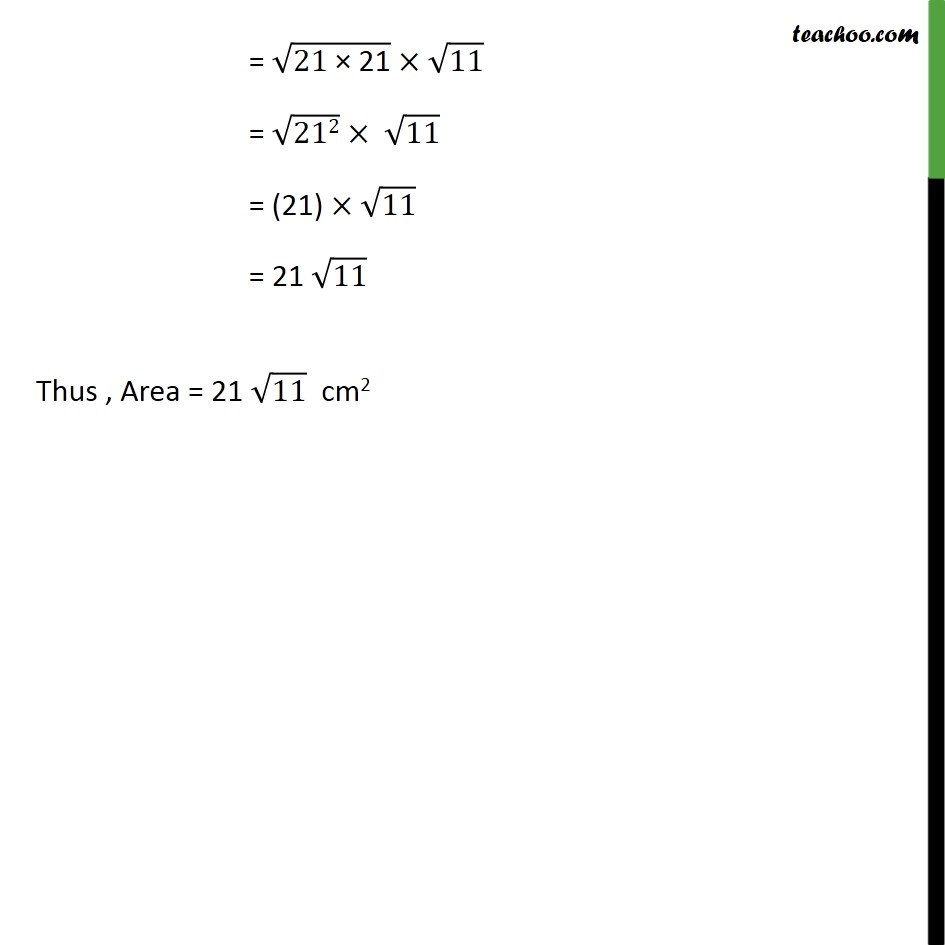Finding area of triangle

Chapter 10 Class 9 Herons Formula
Concept wiseLearn in your speed, with individual attention - Teachoo Maths 1-on-1 Class

### Transcript

Ex 10.1, 4 Find the area of a triangle two sides of which are 18 cm and 10 cm and the perimeter is 42 cm. Area of triangle = (s(s a)(s b)(s c)) Here, s is the semi-perimeter, and a, b, c are the sides of the triangle Given a = 18 cm, b = 10 cm and Perimeter = 42 cm Semi-Perimeter = s =Perimeter/2 s = 42/2 s = 21 cm We need to find c Now, Perimeter = 42cm a + b + c = 42 cm 18 cm + 10 cm + c = 42 cm 28 cm + c = 42 cm c = 42 28 cm c = 14 cm Area of triangle = ( ( )( )( )) Putting a = 18 cm, b =10 cm, c = 14 cm & s = 21 cm Area of triangle = (21(21 18)(21 10)(21 14)) = (21(3)(11)(7)) = (21 (7 3)(11)) = (21 (21)(11)) = ("21 21" ) 11 = ("21" 2) 11 = (21) 11 = 21 11 Thus , Area = 21 11 cm2